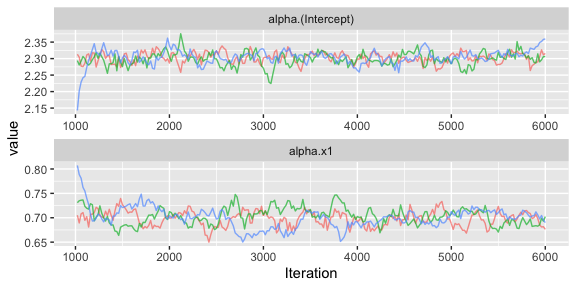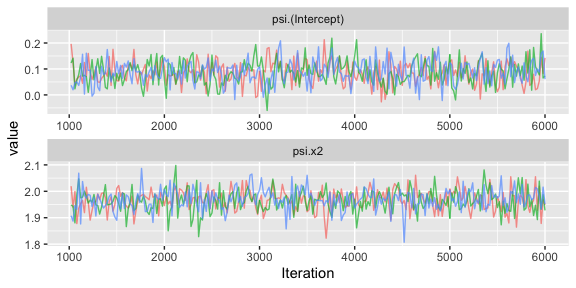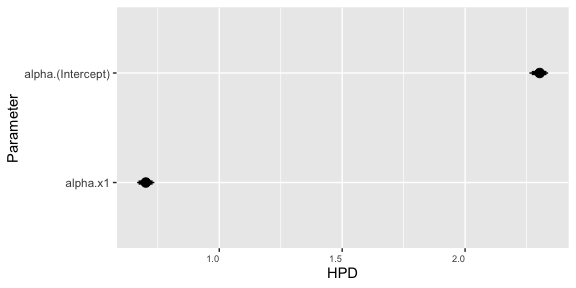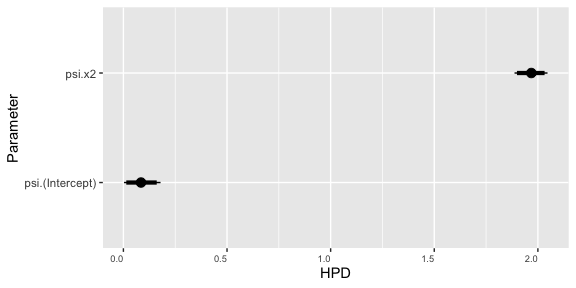# Implementation of Gamma Model

#### 2021-11-22

This package illustrates the implementation of the gamma model for analyzing data including a continuous response that is constrained to be positive. As an example we consider the analysis of a simulated data set. We recommend that you first work through the vignettes for the pied flycatcher data (“pied-flycatchers-1” and “pied-flycatchers-2”) which contain further information on the structure of the package.

# Gamma model

The gamma distribution is parameterized in JAGS according to the shape parameter, $$r$$, and the rate parameter, $$\lambda$$. Given this parametrization the mean and variance of $$Y \sim \mbox{Gamma}(r,\lambda)$$ are $E(Y)=\mu=\frac{r}{\lambda}$ and $\mbox{Var(Y)}=\frac{r}{\lambda^2}=\phi\mu$ where $$\phi=1/\lambda$$ is the dispersion parameter.

In the implementation of the negative binomial double generalized linear mixed effects model the mean and variance for the $$i^{th}$$ observation are modelled as $g(\mu_i)=\eta_i=\mathbf x_{\mu,i}^\top \mathbf \alpha + \mathbf z_{\mu,i}^\top \mathbf \epsilon$ and $h(\phi_i)=\lambda_i= \mathbf x_{\sigma,i}^\top \mathbf \psi + \mathbf z_{\sigma,i}^\top \mathbf \xi$ with $$\mathbf \alpha$$ and $$\mathbf \epsilon$$ representing vectors of fixed and random effects for the mean and $$\mathbf \psi$$ and $$\mathbf \xi$$ fixed and random effects for the dispersion. Both $$\mu_i$$ and $$\phi_i$$ may take any non-negative value, and so it is natural to assume that both $$g(x)=h(x)=log(x)$$, though other link functions may be appropriate in certain situations. The inverse function $$g(x)=1/x$$ is the canonical link for the mean but is not often used in practice because it does not constrain the mean to be positive.

# Library

First you have to load the package:

## Load package
library(dalmatian)

# Raw data

The raw data for this example is provided in the package and can be accessed with:

## Load gamma data
data(gamma_data_1)

This data contains observations from a hypothetical study in which 50 individuals each observed over 30 occasions for which the observed response is a count. Let $$y_{ij}$$ denote observed count on the $$j$$th occasion for individual $$i$$. The counts for each individual are assumed to be drawn from separate, independent, gamma distributions with mean $\mbox{log}(\mu_{i})=\alpha_0 + \alpha_1 x_{i1} + \epsilon_{i}$ where $$x_{i1}$$ and $$z_{i1}$$ represent individual fixed and random effects on the mean and dispersion parameter defined by $\mbox{log}(\phi_{i})=\psi_0 + \psi_1 x_{i2} + \xi_{i}$ where $$x_{i2}$$ and $$\xi_{i}$$ represent individual fixed and random effects on the dispersion. For the simulation we set $$\alpha_0=\log(10)$$, $$\alpha_1=\log(2)$$, $$\psi_0=0$$, and $$\psi_1=2$$. We then generate the fixed and random effects as $x_{i1} \sim \mbox{Normal}(0,1)$ $x_{i2} \sim \mbox{Normal}(0,1)$ $\epsilon_{i} \sim \mbox{Normal}(0,1)$ $\xi_{i} \sim \mbox{Normal}(0,1).$

# Defining the Model

To illustrate the gamma we fit the data generating model to the simulated data. The model structure is defined through two lists specifying the fixed and random effects for the mean and dispersion components:

## Define mean and variance objects
mymean <- list(fixed = list(name = "alpha",
formula = ~x1,
priors = list(c("dnorm",0,.001))),
random = list(name = "epsilon",
formula = ~ID - 1),

mydisp <- list(fixed = list(name = "psi",
formula = ~x2,
priors = list(c("dnorm",0,.001))),
random = list(name = "xi",
formula = ~ID - 1),
link = "log")

# Running the Model with dalmatian

Once the model structure has been defined the model can be fit with the function dalmatian. The following code creates the lists of arguments supplied to jags.model and coda.samples and then calls dalmatian to run the MCMC sampler. Note that the gamma model is fit by specifying that family="gamma".

## Set working directory
## By default uses a system temp directory. You probably want to change this.
workingDir <- tempdir()

## Define list of arguments for jags.model()
jm.args <- list(file=file.path(workingDir,"gamma_test_1.R"),n.chains = 3, n.adapt = 1000)

## Define list of arguments for coda.samples()
cs.args <- list(n.iter=5000,thin=20)

## Run the model using dalmatian
gmcmc <- dalmatian(df=gamma_data_1,
family = "gamma",
mean.model=mymean,
dispersion.model=mydisp,
jags.model.args=jm.args,
coda.samples.args=cs.args,
response = "y",
residuals = FALSE,
run.model = TRUE,
engine = "JAGS",
n.cores = 3,
overwrite = TRUE,
saveJAGSinput = workingDir)

# Results

Once the samples have been generated the post-processing functions can be used to examine the behaviour of the sampler and compute posterior summary statistics. These functions are described further in the help pages and in the vignettes analyzing the pied flycatcher data.

## Convergence Diagnostics

## Compute convergence diagnostics
gconvergence <- convergence(gmcmc)
## Gelman-Rubin diagnostics
gconvergence$gelman ## Potential scale reduction factors: ## ## Point est. Upper C.I. ## alpha.(Intercept) 1.04 1.09 ## alpha.x1 1.03 1.08 ## psi.(Intercept) 1.00 1.00 ## psi.x2 1.00 1.01 ## sd.epsilon.ID 1.00 1.00 ## sd.xi.ID 1.00 1.00 ## Raftery diagnostics gconvergence$raftery
##
## Quantile (q) = 0.025
## Accuracy (r) = +/- 0.005
## Probability (s) = 0.95
##
## You need a sample size of at least 3746 with these values of q, r and s
## Effective sample size
gconvergence$effectiveSize ## alpha.(Intercept) alpha.x1 psi.(Intercept) psi.x2 ## 160.37556 73.53733 680.89428 673.93768 ## sd.epsilon.ID sd.xi.ID ## 372.86996 666.56647 ## Traceplots ## Generate traceplots ## gtraceplots <- traceplots(gmcmc,show=FALSE) ## Fixed effects for mean gtraceplots$meanFixed## Fixed effects for dispersion
gtraceplots$dispersionFixed## Numerical Summaries ## Compute numerical summaries gsummary <- summary(gmcmc) ## Print numerical summaries gsummary ## ## Iterations = 1020:6000 ## Thinning interval = 20 ## Number of chains = 3 ## Sample size per chain = 250 ## ## Posterior Summary Statistics for Each Model Component ## ## Mean Model: Fixed Effects ## Mean Median SD Lower 95% Lower 50% Upper 50% Upper 95% ## (Intercept) 2.3 2.3 0.02 2.26 2.29 2.32 2.34 ## x1 0.7 0.7 0.02 0.67 0.70 0.72 0.74 ## ## Mean Model: Random Effects ## Mean Median SD Lower 95% Lower 50% Upper 50% Upper 95% ## ID 0.1 0.1 0.02 0.07 0.09 0.11 0.13 ## ## Dispersion Model: Fixed Effects ## Mean Median SD Lower 95% Lower 50% Upper 50% Upper 95% ## (Intercept) 0.09 0.08 0.04 0.00 0.06 0.12 0.18 ## x2 1.97 1.97 0.04 1.89 1.95 1.99 2.05 ## ## Dispersion Model: Random Effects ## Mean Median SD Lower 95% Lower 50% Upper 50% Upper 95% ## ID 0.13 0.13 0.06 0.01 0.09 0.18 0.24 ## Graphical Summaries ## Generate caterpillar gcaterpillar <- caterpillar(gmcmc,show = FALSE) ## Fixed effects for mean gcaterpillar$meanFixed## Fixed effects for dispersion
gcaterpillar\$dispersionFixed